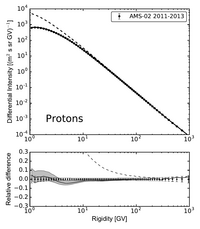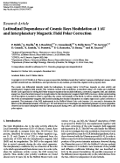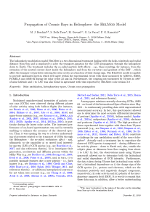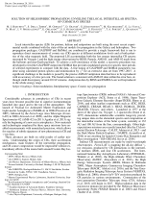HelMod-4 The Heliospheric Modulation Model Online Calculator Version TestCosmic rays diffusion through the interplanetary medium is described by a diffusion tensor ($$K_{ij}$$) that, in a reference system co-moving with the IMF irregularities, can be parametrized as [see e.g. Jokipii 1971 and reference therein]:
\begin{equation}\label{eq:Kmag}
K_{ij}=\left [\begin{array}{ccc}
K_{||} &   0     & 0 \\
0      & K_{\perp,2} & K_A  \\
0           & -K_A           & K_{\perp,3}
\end{array}\right ]
\end{equation}
with $$K_{||}$$ the diffusion coefficient describing the diffusion parallel to the average magnetic field;  $$K_{\perp,3}=K_{\perp,r}$$ and
$$K_{\perp,2}=K_{\perp,\theta}$$ are the diffusion coefficient describing the diffusion perpendicular to the average magnetic field in the radial and polar directions respectively, and finally $$K_A$$ is the so-called  antisymmetric diffusion coefficient described the Magnetic drift across the large scale magnetic field.

A complete parametrization of diffusion coefficients from low to high rigidities is still an open question, but it is common accepted that:
a) at higher rigidity the diffusion coefficient should have a quasi-linear dependence, and b) the diffusion coefficient should be more flat'' at lower rigidity [see, e.g., Palmer 1982].
From HelMod version 3.0 (Boschini et al 2017a,Boschini et al 2017b), for rigidity greater than 1 GV, we use:
\begin{equation}\label{EQ::KparActual}
K_{||}=\frac{\beta}{3} K_0\left( \frac{P}{1\text{GV}}+g_{low}(t)\right) \left(1+\frac{r}{\text{1 AU}}\right),
\end{equation}
where  $$K_0$$ is the diffusion parameter - described in Section 2.1 of Bobik et Al 2012 -, which depends on solar activity and polarity, $$\beta$$ is the particle speed in unit of speed of light, $$P=qc/|Z|e$$ is the particle rigidity expressed in GV, $$r$$ is the heliocentric distance from the Sun in AU and, finally, $$g_{low}$$ is a constant that describes the smoothness of the transition and decrease to 0 during high activity periods.
In present model the spatial dependence is consistent with the one used in Bobik et al 2013 to describe CR latitudinal gradients and shows a radial dependence proportional to $$r$$, but no latitudinal dependence.
The perpendicular diffusion coefficient is taken to be proportional to $$K_{||}$$ with a ratio
$$K_{\perp,i}/K_{||}=\rho_i$$ for both $$r$$ and $$\theta$$ $$i$$-coordinates.

#### BibliographyBobik, P., Boella, G., Boschini, M.~J., Consolandi, C., Della Torre, S., Gervasi, M., Grandi, D., Kudela, K., Pensotti, S., Rancoita P.G. and Tacconi, M. (2012). Astrophys. J., 745:132 .Bobik, P., Boella, G., Boschini, M.~J., Consolandi, C., Della Torre, S., Gervasi, M., Grandi, D., Kudela, K., Pensotti, S., Rancoita P.G., Rozza, D. and Tacconi, M. (2013). Advances in Astronomy, vol. 2013, id.793072Boschini, M.J., Della Torre, S., Gervasi, M., La Vacca, G. and Rancoita, P. G. (2017a)  Accepted for publication in Advances in Space Research. ArXiv:1704.03733, DOI: 10.1016/j.asr.2017.04.017Boschini, M.J., Della Torre, S., Gervasi, M., Grandi, D., Johannesson, G., Kachelriess, M., La Vacca, G., Masi, N., Moskalenko, I. V., Orlando, E., Ostapchenko, S.S., Pensotti, S., Porter, T. A., Quadrani, L., Rancoita, P. G., Rozza, D. and Tacconi, M. (2017b)  Astrophysical Journal, 840:115. Jokipii, J. R. (1971), Reviews of Geophysics and Space Physics, Vol. 9, p. 27 - 87. Palmer, I. D. (1982), Reviews of Geophysics and Space Physics, vol. 20, p. 335-351.